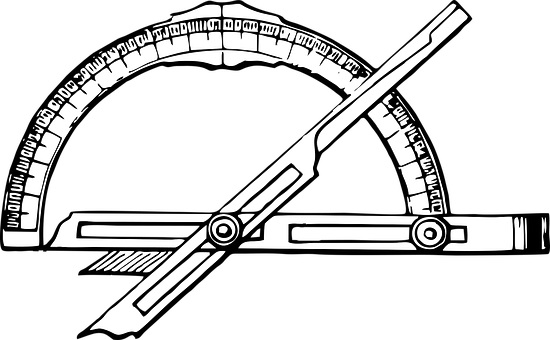## Angle Subtended by a Chord of Circle Class 10th

Introduction In a circle, if we draw a chord and join the two ends of the chord to the third point which is situated at the Centre or on the circle. Then the angle made at the third point is the angle subtended by the chord of the Circle. In the above figure, ∠PRQ and …